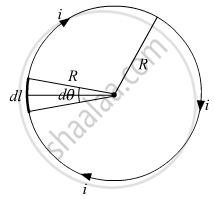Advertisement Remove all ads

# State and explain the law used to determine the magnetic field at a point due to a current element. Derive the expression for the magnetic field due to a circular current-carrying loop - Physics

Advertisement Remove all ads
Advertisement Remove all ads
Advertisement Remove all ads
Answer in Brief

State and explain the law used to determine the magnetic field at a point due to a current element. Derive the expression for the magnetic field due to a circular current-carrying loop of radius r at its center.

Advertisement Remove all ads

#### Solution

The magnetic field at a certain point due to an element of a current-carrying conductor is given by Biot Savart's law. According to Biot-Savart’s law, the magnitude of the magnetic field dB due to a current-carrying element of length |dl| at a distance r is proportional to the current I, length of the element |dl|, and inversely proportional to the square of the distance r. Its direction is perpendicular to the plane containing dl and r.

Thus, in vector notation,

 "dB"  alpha  (vec("idl") xx vec("r"))/"r"^3

"dB" = mu_° /(4pi) (vec("idl") xx "r")/"r"^3

Magnetic field due to a circular current carrying loop:Let the current in the circular loop of radius R is i, now if take a small element of the loop, dl, making an angle dθ at the center.

The magnetic field at the center by using Biot-Savart law is, vec"dB" = mu_°/(4pi) (vec"idl" xx vec"R")/("R"^3) = mu_°/(4pi) ("idl")/"R"^2 hat"n" ...(vec"dl" ⊥ vec"R", hat"n" ⊥ vec "dl"& vec"R")

∵ dl = rdθ

⇒ |vec"dB"| = mu_° /(4pi) ("Rd"theta)/R^2 = (mu_°"i")/(4pi"R")"d"theta

⇒ |vec"B"| = mu_° /(4pi"R") int_0^(2pi) "d"theta = (mu_°"i")/(2"R")

⇒ |vec"B"| = (mu_°"i")/(2"R")

⇒ vec"B" = (mu_°"i")/(2"R") hat"n" ....(Where hat"n" is a vector perpendicular to the plane of loop, directed downward)

Concept: Magnetic Field Due to a Current Element, Biot-Savart Law
Is there an error in this question or solution?

#### Video TutorialsVIEW ALL 

Advertisement Remove all ads
Share
Notifications

View all notifications

Forgot password?